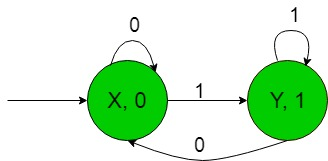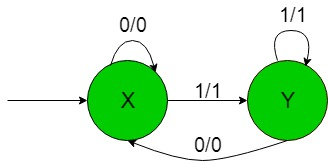# TOC | Construction of the machines to produce residue modulo ‘2’ of binary numbers

Prerequisite: Mealy and Moore Machines, Difference between Mealy machine and Moore machine
In this article, we will see some designing of Finite Automata with Output i.e, Moore and Mealy machines.

Problem: Construction of the machines that take the binary number {0, 1} as input and produce residue modulo ‘2’ as output i.e, when the equivalent decimal number of binary input over {0, 1} is divided by 2 then it gives output as it’s remainder.
Assume,

```Ε = {0, 1} and
Δ = {0, 1} ```

where Ε and Δ are the input and output alphabet respectively.

The required Moore machine is constructed below:In the above diagram, the initial state ‘X’ on getting ‘0’ as the input it remains in the state of itself and prints ‘0’ as the output and on getting ‘1’ as the input it transmits to a state ‘Y’ and prints ‘1’ as the output so on for the remaining states.

For example, when the input string is ’10’ then above Moore machine produce 0 as the output because the decimal equivalent of binary input ’10’ is 2 and 2 divided by 2 is 0 i.e, the remainder is 0. Thus finally above Moore machine can easily produce residue modulo ‘2’ as output i.e, when the equivalent decimal number of binary input over {0, 1} is divided by 2 then it gives output as it’s remainder.

The required Mealy machine is constructed below:In the above diagram, the initial state ‘X’ on getting ‘0’ as the input it remains in the state of itself and prints ‘0’ as the output and on getting ‘1’ as the input it transmits to a state ‘Y’ and prints ‘1’ as the output. The state ‘Y’ on getting ‘1’ as the input it remains in the state of itself and prints ‘1’ as the output and on getting ‘0’ as the input it goes back to the initial state ‘X’ and prints ‘0’ as the output.

For example, when the input string is ’10’ then above Mealy machine produce 0 as the output because the decimal equivalent of binary input ’10’ is 2 and 2 divided by 2 is 0 i.e, the remainder is 0. Thus finally above Mealy machine can easily produce residue modulo ‘2’ as output i.e, when the equivalent decimal number of binary input over {0, 1} is divided by 2 then it gives output as it’s remainder.My Personal Notes arrow_drop_upCheck out this Author's contributed articles.

If you like GeeksforGeeks and would like to contribute, you can also write an article using contribute.geeksforgeeks.org or mail your article to contribute@geeksforgeeks.org. See your article appearing on the GeeksforGeeks main page and help other Geeks.

Please Improve this article if you find anything incorrect by clicking on the "Improve Article" button below.

Article Tags :

Be the First to upvote.

Please write to us at contribute@geeksforgeeks.org to report any issue with the above content.